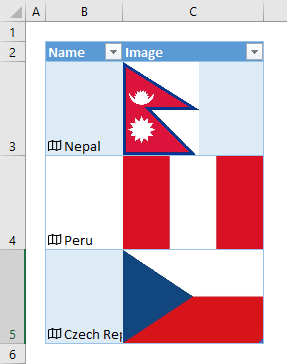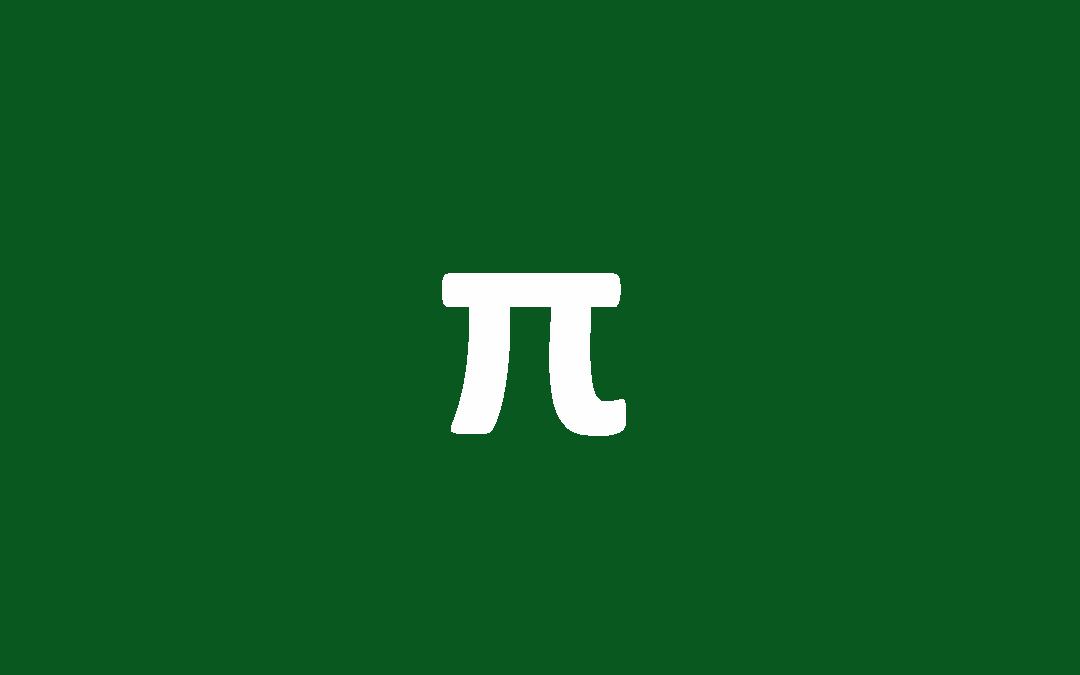## How to create slopegraphs in Excel

Excel doesn’t have a predefined chart category for slopegraphs, but you can modify a line chart to create one. In this guide, we’re going to show you how to create slopegraphs in Excel. Download Workbook What is a slopegraph? Slopegraphs are good for displaying...## How to insert country flags in Excel

In this guide, we’re going to show you how to insert country flags in Excel. Download Workbook There are two ways of inserting flags into your Excel workbook. First is to find the images and insert them into your workbook copy pasting. If you place the flags...## PI Function in Excel and Everything You Need to Know About It

The PI function is a Math and Trigonometry formula that returns the equivalent value for the mathematical constant pi (π), which is commonly used in finding the diameter or volume of circles and spherical objects. In this guide, we’re going to show you how to use the...## Treemap Excel Charts: The Perfect Tool for Displaying Hierarchical Data

A treemap chart is a type of data visualization that is especially useful for displaying hierarchical data. On a treemap, each item is represented by a rectangular shape, where smaller rectangles represent the sub-groups. The color and size of rectangles are typically...

## Excel CONVERT Function Units

Weight and Mass Weight and mass From_unit or to_unit Gram “g” Slug “sg” Pound mass (avoirdupois) “lbm” U (atomic mass unit) “u” Ounce mass (avoirdupois) “ozm” Grain “grain” U.S. (short)...